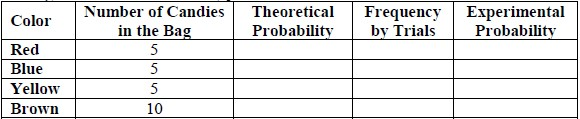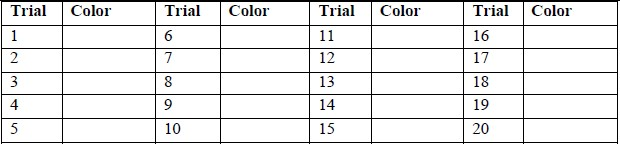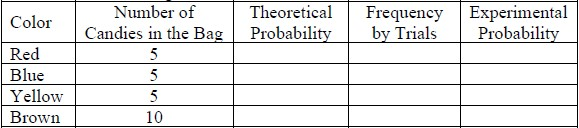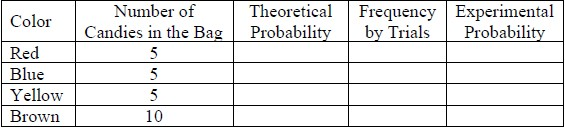# MA.7.DP.2.4Export Print
Use a simulation of a simple experiment to find experimental probabilities and compare them to theoretical probabilities.

### Examples

Investigate whether a coin is fair by tossing it 1,000 times and comparing the percentage of heads to the theoretical probability 0.5.

### Clarifications

Clarification 1: Instruction includes representing probability as a fraction, percentage or decimal.

Clarification 2: Instruction includes recognizing that experimental probabilities may differ from theoretical probabilities due to random variation. As the number of repetitions increases experimental probabilities will typically better approximate the theoretical probabilities.

Clarification 3: Experiments include tossing a fair coin, rolling a fair die, picking a card randomly from a deck, picking marbles randomly from a bag and spinning a fair spinner.

General Information
Subject Area: Mathematics (B.E.S.T.)
Strand: Data Analysis and Probability
Status: State Board Approved

## Benchmark Instructional Guide

### Terms from the K-12 Glossary

• Event
• Experimental Probability
• Simulation
• Theoretical Probability

### Vertical Alignment

Previous Benchmarks

Next Benchmarks

### Purpose and Instructional Strategies

In grade 7, students use a simulation of a simple experiment to find experimental probabilities and compare them to theoretical probabilities. In grade 8, students will solve real-world problems involving probabilities related to single or repeated experiments, including making predictions based on theoretical probability.
• Instruction includes opportunities for students to run various numbers of trials to discover that the increased repetition of the experiment will bring the experimental probability closer to the theoretical. Use virtual simulations to quickly show higher and higher volumes of repetition that would be difficult to create with physical manipulatives (MTR.5.1).
• Remind students that chance has no memory and each repetition in the simulation has the same probability distribution for the possible events.
• For example, if you flip a coin and it lands on heads, the next flip does not rely on the first outcome and still can be either heads or tails.
• Instruction focuses on the simple experiments listed in Clarification 3.
• For example, students can roll a 6-sided die 30 times to determine the experimental probability of “not rolling a 2.” Students can then compare their experimental probability to the theoretical probability of “not rolling a 2,” which is $\frac{\text{5}}{\text{6}}$.

### Common Misconceptions or Errors

• Students may incorrectly assume the theoretical and experimental probabilities of the same experiment will always be the same. To address this misconception, provide multiple opportunities for students to experience simulations of different situations, with physical or virtual manipulatives, in order to find and compare the experimental and theoretical probabilities.
• Students may incorrectly expect to see every possible outcome occur during a simulation. While all may occur in a simulation, it is not certain to happen. Students may inadvertently let their own experience with an experiment affect their response.
• For example, during an experiment if a student never draws an ace from a standard deck of cards, this does not indicate it could never happen.

### Strategies to Support Tiered Instruction

• Teacher reviews the root words theoretical (theory) and experimental (experiment) and discusses the difference between a theoretical probability and experimental probability. Teacher provides graphic organizer to keep as reference for root words.
• For example, experimental probabilities are from simulations whereas theoretical probabilities are from calculations.
• Teacher provides opportunities to see a variety of outcomes.
• For example, open a deck of cards and draw 5 random cards. After looking at the 5 cards, discuss all the possible cards that could have been drawn but were not. This will help students see that not all possible outcomes will occur when an experiment is done.
• Teacher provides multiple examples for students to discuss if a probability in the example is theoretical or experimental. After each answer, students discuss how they know it is theoretical or experimental.
• For example, if one tosses a fair coin, the theoretical probability of landing on heads is 0.5. If one tosses a fair coin 14 times and it lands on hands 9 times, the experimental probability of landing on heads is $\frac{\text{9}}{\text{14}}$ based on the simulation.
• Teacher provides multiple opportunities for students to experience simulations of different situations, with physical or virtual manipulatives, in order to find and compare the experimental and theoretical probabilities.

Each set of partners has been given a bag containing 5 red, 5 green, 5 yellow and 10 brown candies.
• Part A. Determine the theoretical probability for selecting one red candy at random from the bag. Do the same for blue, yellow and brown.• Part B. Experimental Trials: Select one candy from the bag, record its color in the table below and return it to the bag. Repeat this process for a total of 20 trials.• Part C. In the original table, record the total frequency of each color based on your 20 trials. Then calculate the experimental probability for each. How do the theoretical and experimental probabilities compare?
• Part D. Collect the data from 2 other sets of partners and combine your total frequencies. Complete the table below based on those 60 trials. How do the theoretical and experimental probabilities compare? How does that compare to your original calculations using 20 trials?• Part E. Collect all of the class data to calculate new total frequencies and complete the table below.• Part F.
• How do the theoretical and experimental probabilities compare?
• How does that compare to the calculations using 20 trials and 60 trials?
• What conclusions can you make about theoretical and experimental probabilities based on this information?

### Instructional Items

Instructional Item 1
A bag contains green marbles and purple marbles. If a marble is randomly selected from the bag, the probability that it is green is 0.6 and the probability that it is purple is 0.4. Dylan draws a marble from the bag, notes its color, and returns it to the bag. He does this 50 times. Approximately, how many times would you expect Dylan to draw a green marble?

*The strategies, tasks and items included in the B1G-M are examples and should not be considered comprehensive.

## Related Courses

This benchmark is part of these courses.
1200400: Foundational Skills in Mathematics 9-12 (Specifically in versions: 2014 - 2015, 2015 - 2022, 2022 and beyond (current))
1205020: M/J Accelerated Mathematics Grade 6 (Specifically in versions: 2014 - 2015, 2015 - 2020, 2020 - 2022, 2022 and beyond (current))
1205040: M/J Grade 7 Mathematics (Specifically in versions: 2014 - 2015, 2015 - 2022, 2022 and beyond (current))
1204000: M/J Foundational Skills in Mathematics 6-8 (Specifically in versions: 2014 - 2015, 2015 - 2022, 2022 and beyond (current))
7812020: Access M/J Grade 7 Mathematics (Specifically in versions: 2014 - 2015, 2015 - 2018, 2018 - 2019, 2019 - 2022, 2022 and beyond (current))

## Related Access Points

Alternate version of this benchmark for students with significant cognitive disabilities.
MA.7.DP.2.AP.4: Conduct a simple experiment to find experimental probabilities.

## Related Resources

Vetted resources educators can use to teach the concepts and skills in this benchmark.

## Formative Assessments

Hen Eggs:

Students are asked to estimate the probability of a chance event based on observed frequencies.

Type: Formative Assessment

Marble Probability:

Students are asked to determine probabilities based on observed outcomes and to determine if the outcomes appear to be equally likely.

Type: Formative Assessment

Number Cube:

Students are asked to determine probabilities based on observed outcomes and to determine if the outcomes appear to be equally likely.

Type: Formative Assessment

Errand Runner:

Students are asked to determine the probability of a chance event and explain possible causes for the difference between the probability and observed frequencies.

Type: Formative Assessment

## Lesson Plans

Genetics can be a Monster!:

In this lesson, students will use Punnett squares to calculate the probabilities of different genotypes and phenotypes produced by genetic crosses.

Type: Lesson Plan

Computer Simulated Experiments in Genetics:

A computer simulation package called "Star Genetics" is used to generate progeny for one or two additional generations. The distribution of the phenotypes of the progeny provide data from which the parental genotypes can be inferred. The number of progeny can be chosen by the student in order to increase the student's confidence in the inference.

Type: Lesson Plan

## Perspectives Video: Experts

How Math Models Help Insurance Companies After a Hurricane Hits:

Hurricanes can hit at any time! How do insurance companies use math and weather data to help to restore the community?

Type: Perspectives Video: Expert

Probabilistic Weather Modeling:

Meteorologist from Risk Management discusses the use of probability in predicting hurricane tracks.

Type: Perspectives Video: Expert

Let's Make a Math Deal:

Should I keep my choice or switch?  Learn more about the origins and probability behind the Monty Hall door picking dilemma and how Game Theory and strategy effect the probability.

Type: Perspectives Video: Expert

## Text Resource

Shuffling Shenanigans:

This informational text resource is intended to support reading in the content area. A student in love with magic card tricks asks and answers his own math questions after pursuing a career as a mathematician in order to solve them. How many times must a deck be shuffled to achieve a truly random mix of cards? The answer lies within.

Type: Text Resource

## Tutorials

Comparing Theoretical to Experimental Probabilites:

This video compares theoretical and experimantal probabilities and sources of possible discrepancy.

Type: Tutorial

Making Predictions with Probability:

Watch the video as it predicts the number of times a spinner will land on a given outcome.

Type: Tutorial

## MFAS Formative Assessments

Errand Runner:

Students are asked to determine the probability of a chance event and explain possible causes for the difference between the probability and observed frequencies.

Hen Eggs:

Students are asked to estimate the probability of a chance event based on observed frequencies.

Marble Probability:

Students are asked to determine probabilities based on observed outcomes and to determine if the outcomes appear to be equally likely.

Number Cube:

Students are asked to determine probabilities based on observed outcomes and to determine if the outcomes appear to be equally likely.

## Student Resources

Vetted resources students can use to learn the concepts and skills in this benchmark.

## Tutorials

Comparing Theoretical to Experimental Probabilites:

This video compares theoretical and experimantal probabilities and sources of possible discrepancy.

Type: Tutorial

Making Predictions with Probability:

Watch the video as it predicts the number of times a spinner will land on a given outcome.

Type: Tutorial

## Parent Resources

Vetted resources caregivers can use to help students learn the concepts and skills in this benchmark.